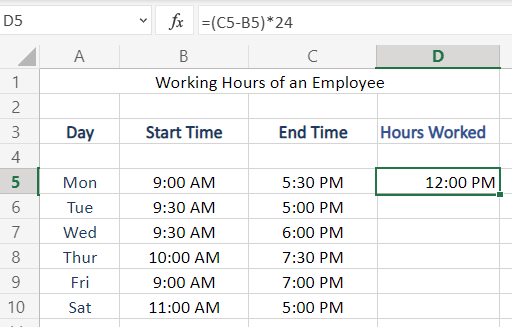# Calculate Time Difference in Excel (Subtract time)There are many use case scenarios where we need to find the difference between times. For example, when we need to calculate working hours, study hours, and anything as such.

Although we can always calculate time difference in Excel manually or by using a calculator, this would be a very tiresome and lengthy procedure when considering a large data set.

## Steps to calculate Time Difference in Excel

Calculating Time Difference in Excel cannot be performed by simple subtraction operation (-). Let’s take a look at how exactly we can calculate time difference in Excel.

### Step 1. Input the time data

• Enter the data in your Excel worksheet.

The data should be in time format, as for calculating a difference between two times.

Our sample data below is to calculate the total working hours of an employee in a week, where the start time and end time of the Employee’s working are recorded in an excel spreadsheet.

### Step 2. Perform formula on the dates

• Apply subtraction (—) operation,
• Subtract Start Time from End Time.
• To apply, type in = sign followed by brackets.
• In the brackets apply subtraction operation like: End Time – Start Time

here, in our example C5-B5

• Now, as we are dealing with time and need to apply 24 hour time formatting to get our result in hours,
• Multiply (*) the subtraction result by 24 to apply 24 hour formatting.Multiply The Result By 24, for Hour Formatting of time

But this is not the result of the number of hours between 9:00 am to 5:30 pm as per our example, if we calculate manually. Follow the below step to render this formatting issue.

### Step 3. Apply Comma Formatting

• You might have guessed, that you have performed something erroneous by watching the result. But don’t worry, everything is fine.
• You just need to apply comma formatting to your result now, to apply Comma Formatting, select the cell of previous step result and use the shortcut key:

Ctrl + Shift + !

By now you must have got the correct result after applying the comma formatting to the result of step 2.

Apply the same to all the remaining cells, by dragging the bottom right option button that occurs at the border when selecting that particular cell.

This is how we calculate time difference in Excel.

But as for our example, here we calculate the total working hours of an employee in a week:

We calculate, the total hours in a week by applying SUM function to the hours worked column.